Problem
A baseball diamond is 90 feet square, and the pitcher's mound is at the center of the square. If a pitcher throws a baseball at 100 miles per hour, how fast is the distance between the ball and first base changing as the ball crosses home plate?
Solution
We draw a diagram and fill in the relevant information.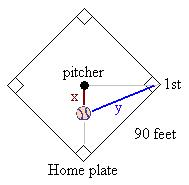x = distance from pitcher to ball
y = distance from first base to ball
distance from first base to pitcher =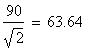approximately
In mathematical terms, we want to find dy/dt when the ball is at home plate. We know the velocity of the ball as it travels away from the pitcher is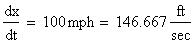The Pythagorean Theorem gives us a relationship between x and y: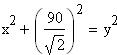Differentiate implicitly with respect to t to get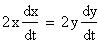Substitute what we know and solve for dy/dt.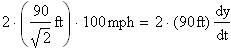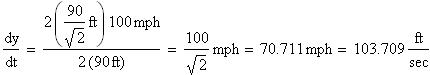So when the ball traveling 100 miles per hour reaches home plate, the distance between the ball and first base is increasing at about 71 miles per hour, or about 104 feet per second.